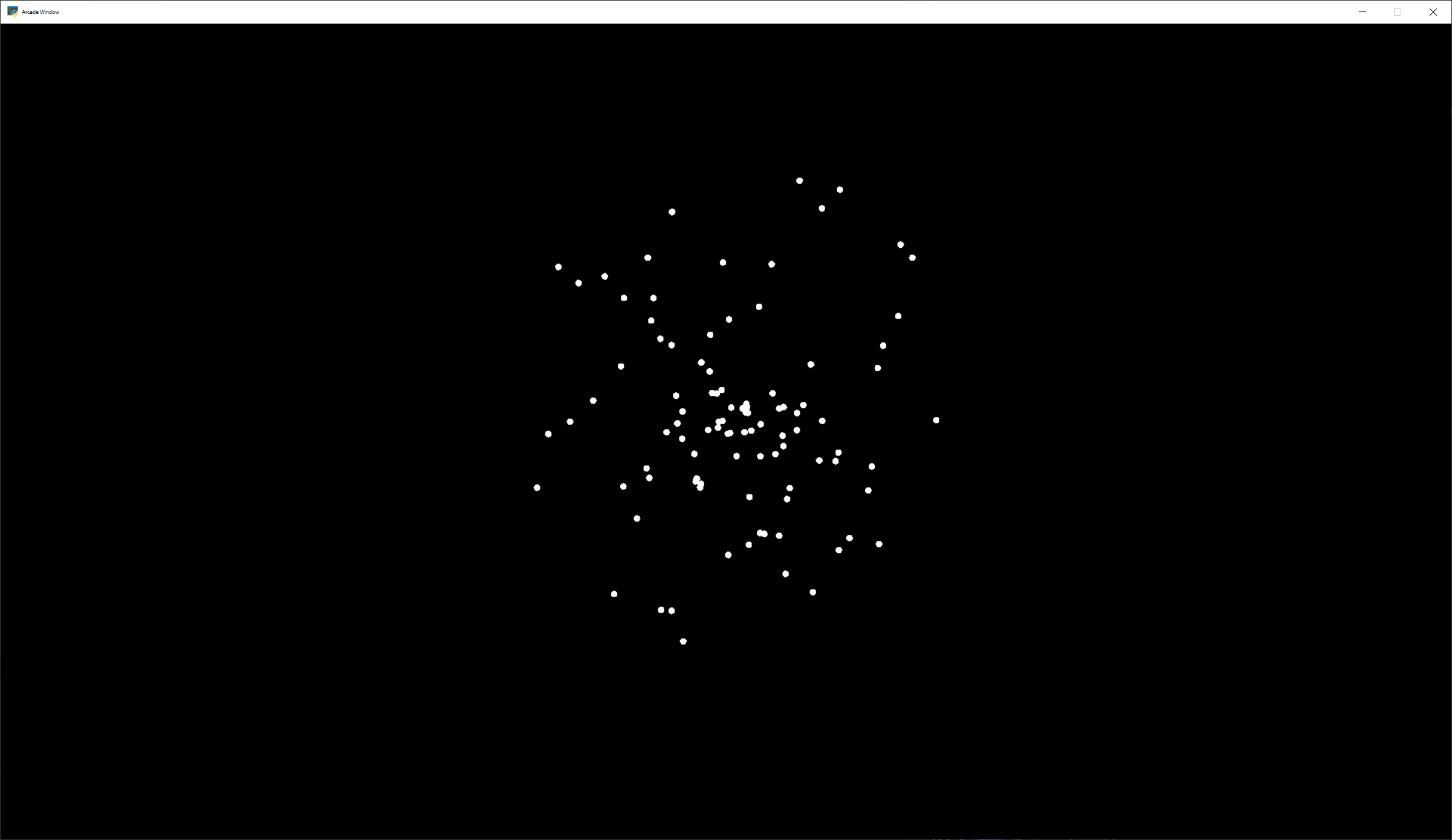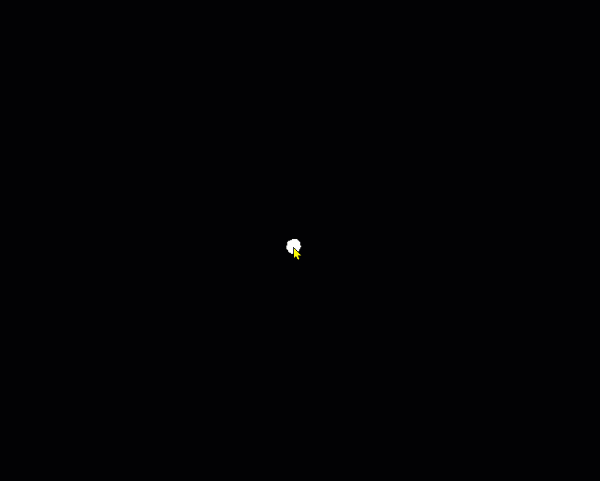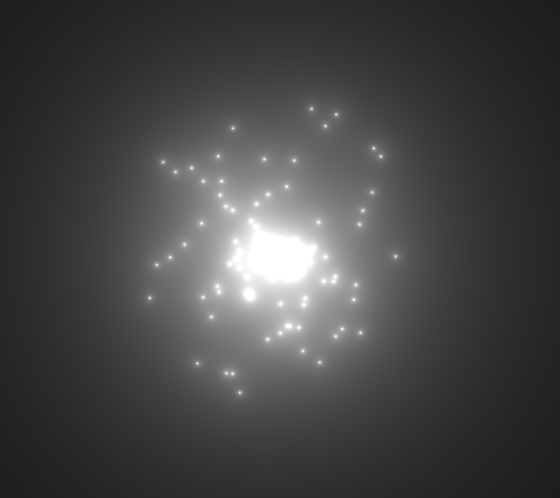# Shader Toy Tutorial - Particles#

This tutorial assumes you are already familiar with the material in Shader Toy Tutorial - Glow. In this tutorial, we take a look at adding animated particles. These particles can be used for an explosion effect.

The “trick” to this example, is the use of pseudo-random numbers to generate each particle’s angle and speed from the initial explosion point. Why “pseudo-random”? This allows each processor on the GPU to independently calculate each particle’s position at any point and time. We can then allow the GPU to calculate in parallel.

First, we need a program that will load a shader. This program is also keeping track of how much time has elapsed. This is necessary for us to calculate how far along the animation sequence we are.

 ``` 1 2 3 4 5 6 7 8 9 10 11 12 13 14 15 16 17 18 19 20 21 22 23 24 25 26 27 28 29 30 31 32 33 34 35 36``` ```import arcade from arcade.experimental import Shadertoy # Derive an application window from Arcade's parent Window class class MyGame(arcade.Window): def __init__(self): # Call the parent constructor super().__init__(width=1920, height=1080) # Used to track run-time self.time = 0.0 # Load a file and create a shader from it file_name = "explosion.glsl" self.shadertoy = Shadertoy(size=self.get_size(), main_source=open(file_name).read()) def on_draw(self): self.clear() # Set uniform data to send to the GLSL shader self.shadertoy.program['pos'] = self.mouse["x"], self.mouse["y"] # Run the GLSL code self.shadertoy.render(time=self.time) def on_update(self, delta_time: float): # Track run time self.time += delta_time if __name__ == "__main__": window = MyGame() window.center_window() arcade.run() ```

## Initial shader with particles#``` 1 2 3 4 5 6 7 8 9 10 11 12 13 14 15 16 17 18 19 20 21 22 23 24 25 26 27 28 29 30 31 32 33 34 35 36 37 38 39 40 41 42 43 44 45 46 47 48 49 50 51 52 53``` ```// Origin of the particles uniform vec2 pos; // Constants // Number of particles const float PARTICLE_COUNT = 100.0; // Max distance the particle can be from the position. // Normalized. (So, 0.3 is 30% of the screen.) const float MAX_PARTICLE_DISTANCE = 0.3; // Size of each particle. Normalized. const float PARTICLE_SIZE = 0.004; const float TWOPI = 6.2832; // This function will return two pseudo-random numbers given an input seed. // The result is in polar coordinates, to make the points random in a circle // rather than a rectangle. vec2 Hash12_Polar(float t) { float angle = fract(sin(t * 674.3) * 453.2) * TWOPI; float distance = fract(sin((t + angle) * 724.3) * 341.2); return vec2(sin(angle), cos(angle)) * distance; } void mainImage( out vec4 fragColor, in vec2 fragCoord ) { // Normalized pixel coordinates (from 0 to 1) // Origin of the particles vec2 npos = (pos - .5 * iResolution.xy) / iResolution.y; // Position of current pixel we are drawing vec2 uv = (fragCoord- .5 * iResolution.xy) / iResolution.y; // Re-center based on input coordinates, rather than origin. uv -= npos; // Default alpha is transparent. float alpha = 0.0; // Loop for each particle for (float i= 0.; i < PARTICLE_COUNT; i++) { // Direction of particle + speed float seed = i + 1.0; vec2 dir = Hash12_Polar(seed); // Get position based on direction, magnitude, and explosion size vec2 particlePosition = dir * MAX_PARTICLE_DISTANCE; // Distance of this pixel from that particle float d = length(uv - particlePosition); // If we are within the particle size, set alpha to 1.0 if (d < PARTICLE_SIZE) alpha = 1.0; } // Output to screen fragColor = vec4(1.0, 1.0, 1.0, alpha); } ```

## Add particle movement#``` 1 2 3 4 5 6 7 8 9 10 11 12 13 14 15 16 17 18 19 20 21 22 23 24 25 26 27 28 29 30 31 32 33 34 35 36 37 38 39 40 41 42 43 44 45 46 47 48 49 50 51 52 53 54 55 56 57 58 59 60``` ```// Origin of the particles uniform vec2 pos; // Constants // Number of particles const float PARTICLE_COUNT = 100.0; // Max distance the particle can be from the position. // Normalized. (So, 0.3 is 30% of the screen.) const float MAX_PARTICLE_DISTANCE = 0.3; // Size of each particle. Normalized. const float PARTICLE_SIZE = 0.004; // Time for each burst cycle, in seconds. const float BURST_TIME = 2.0; const float TWOPI = 6.2832; // This function will return two pseudo-random numbers given an input seed. // The result is in polar coordinates, to make the points random in a circle // rather than a rectangle. vec2 Hash12_Polar(float t) { float angle = fract(sin(t * 674.3) * 453.2) * TWOPI; float distance = fract(sin((t + angle) * 724.3) * 341.2); return vec2(sin(angle), cos(angle)) * distance; } void mainImage( out vec4 fragColor, in vec2 fragCoord ) { // Normalized pixel coordinates (from 0 to 1) // Origin of the particles vec2 npos = (pos - .5 * iResolution.xy) / iResolution.y; // Position of current pixel we are drawing vec2 uv = (fragCoord- .5 * iResolution.xy) / iResolution.y; // Re-center based on input coordinates, rather than origin. uv -= npos; // Default alpha is transparent. float alpha = 0.0; // 0.0 - 1.0 normalized fraction representing how far along in the explosion we are. // Auto resets if time goes beyond burst time. This causes the explosion to cycle. float timeFract = fract(iTime * 1 / BURST_TIME); // Loop for each particle for (float i= 0.; i < PARTICLE_COUNT; i++) { // Direction of particle + speed float seed = i + 1.0; vec2 dir = Hash12_Polar(seed); // Get position based on direction, magnitude, and explosion size // Adjust based on time scale. (0.0-1.0) vec2 particlePosition = dir * MAX_PARTICLE_DISTANCE * timeFract; // Distance of this pixel from that particle float d = length(uv - particlePosition); // If we are within the particle size, set alpha to 1.0 if (d < PARTICLE_SIZE) alpha = 1.0; } // Output to screen fragColor = vec4(1.0, 1.0, 1.0, alpha); } ```

 ``` 1 2 3 4 5 6 7 8 9 10 11 12 13 14 15 16 17 18 19 20 21 22 23 24 25 26 27 28 29 30 31 32 33 34 35 36 37 38 39 40 41 42 43 44 45 46 47 48 49 50 51 52 53 54 55 56 57 58 59 60``` ```// Origin of the particles uniform vec2 pos; // Constants // Number of particles const float PARTICLE_COUNT = 100.0; // Max distance the particle can be from the position. // Normalized. (So, 0.3 is 30% of the screen.) const float MAX_PARTICLE_DISTANCE = 0.3; // Size of each particle. Normalized. const float PARTICLE_SIZE = 0.004; // Time for each burst cycle, in seconds. const float BURST_TIME = 2.0; const float TWOPI = 6.2832; // This function will return two pseudo-random numbers given an input seed. // The result is in polar coordinates, to make the points random in a circle // rather than a rectangle. vec2 Hash12_Polar(float t) { float angle = fract(sin(t * 674.3) * 453.2) * TWOPI; float distance = fract(sin((t + angle) * 724.3) * 341.2); return vec2(sin(angle), cos(angle)) * distance; } void mainImage( out vec4 fragColor, in vec2 fragCoord ) { // Normalized pixel coordinates (from 0 to 1) // Origin of the particles vec2 npos = (pos - .5 * iResolution.xy) / iResolution.y; // Position of current pixel we are drawing vec2 uv = (fragCoord- .5 * iResolution.xy) / iResolution.y; // Re-center based on input coordinates, rather than origin. uv -= npos; // Default alpha is transparent. float alpha = 0.0; // 0.0 - 1.0 normalized fraction representing how far along in the explosion we are. // Auto resets if time goes beyond burst time. This causes the explosion to cycle. float timeFract = fract(iTime * 1 / BURST_TIME); // Loop for each particle for (float i= 0.; i < PARTICLE_COUNT; i++) { // Direction of particle + speed float seed = i + 1.0; vec2 dir = Hash12_Polar(seed); // Get position based on direction, magnitude, and explosion size // Adjust based on time scale. (0.0-1.0) vec2 particlePosition = dir * MAX_PARTICLE_DISTANCE * timeFract; // Distance of this pixel from that particle float d = length(uv - particlePosition); // If we are within the particle size, set alpha to 1.0 if (d < PARTICLE_SIZE) alpha = 1.0; } // Output to screen fragColor = vec4(1.0, 1.0, 1.0, alpha * (1.0 - timeFract)); } `````` 1 2 3 4 5 6 7 8 9 10 11 12 13 14 15 16 17 18 19 20 21 22 23 24 25 26 27 28 29 30 31 32 33 34 35 36 37 38 39 40 41 42 43 44 45 46 47 48 49 50 51 52 53 54 55 56 57 58 59 60 61 62``` ```// Origin of the particles uniform vec2 pos; // Constants // Number of particles const float PARTICLE_COUNT = 100.0; // Max distance the particle can be from the position. // Normalized. (So, 0.3 is 30% of the screen.) const float MAX_PARTICLE_DISTANCE = 0.3; // Size of each particle. Normalized. const float PARTICLE_SIZE = 0.004; // Time for each burst cycle, in seconds. const float BURST_TIME = 2.0; // Particle brightness const float DEFAULT_BRIGHTNESS = 0.0005; const float TWOPI = 6.2832; // This function will return two pseudo-random numbers given an input seed. // The result is in polar coordinates, to make the points random in a circle // rather than a rectangle. vec2 Hash12_Polar(float t) { float angle = fract(sin(t * 674.3) * 453.2) * TWOPI; float distance = fract(sin((t + angle) * 724.3) * 341.2); return vec2(sin(angle), cos(angle)) * distance; } void mainImage( out vec4 fragColor, in vec2 fragCoord ) { // Normalized pixel coordinates (from 0 to 1) // Origin of the particles vec2 npos = (pos - .5 * iResolution.xy) / iResolution.y; // Position of current pixel we are drawing vec2 uv = (fragCoord- .5 * iResolution.xy) / iResolution.y; // Re-center based on input coordinates, rather than origin. uv -= npos; // Default alpha is transparent. float alpha = 0.0; // 0.0 - 1.0 normalized fraction representing how far along in the explosion we are. // Auto resets if time goes beyond burst time. This causes the explosion to cycle. float timeFract = fract(iTime * 1 / BURST_TIME); // Loop for each particle for (float i= 0.; i < PARTICLE_COUNT; i++) { // Direction of particle + speed float seed = i + 1.0; vec2 dir = Hash12_Polar(seed); // Get position based on direction, magnitude, and explosion size // Adjust based on time scale. (0.0-1.0) vec2 particlePosition = dir * MAX_PARTICLE_DISTANCE * timeFract; // Distance of this pixel from that particle float d = length(uv - particlePosition); // Add glow based on distance alpha += DEFAULT_BRIGHTNESS / d; } // Output to screen fragColor = vec4(1.0, 1.0, 1.0, alpha * (1.0 - timeFract)); } ```
 ``` 1 2 3 4 5 6 7 8 9 10 11 12 13 14 15 16 17 18 19 20 21 22 23 24 25 26 27 28 29 30 31 32 33 34 35 36 37 38 39 40 41 42 43 44 45 46 47 48 49 50 51 52 53 54 55 56 57 58 59 60 61 62 63 64 65``` ```// Origin of the particles uniform vec2 pos; // Constants // Number of particles const float PARTICLE_COUNT = 100.0; // Max distance the particle can be from the position. // Normalized. (So, 0.3 is 30% of the screen.) const float MAX_PARTICLE_DISTANCE = 0.3; // Size of each particle. Normalized. const float PARTICLE_SIZE = 0.004; // Time for each burst cycle, in seconds. const float BURST_TIME = 2.0; // Particle brightness const float DEFAULT_BRIGHTNESS = 0.0005; // How many times to the particles twinkle const float TWINKLE_SPEED = 10.0; const float TWOPI = 6.2832; // This function will return two pseudo-random numbers given an input seed. // The result is in polar coordinates, to make the points random in a circle // rather than a rectangle. vec2 Hash12_Polar(float t) { float angle = fract(sin(t * 674.3) * 453.2) * TWOPI; float distance = fract(sin((t + angle) * 724.3) * 341.2); return vec2(sin(angle), cos(angle)) * distance; } void mainImage( out vec4 fragColor, in vec2 fragCoord ) { // Normalized pixel coordinates (from 0 to 1) // Origin of the particles vec2 npos = (pos - .5 * iResolution.xy) / iResolution.y; // Position of current pixel we are drawing vec2 uv = (fragCoord- .5 * iResolution.xy) / iResolution.y; // Re-center based on input coordinates, rather than origin. uv -= npos; // Default alpha is transparent. float alpha = 0.0; // 0.0 - 1.0 normalized fraction representing how far along in the explosion we are. // Auto resets if time goes beyond burst time. This causes the explosion to cycle. float timeFract = fract(iTime * 1 / BURST_TIME); // Loop for each particle for (float i= 0.; i < PARTICLE_COUNT; i++) { // Direction of particle + speed float seed = i + 1.0; vec2 dir = Hash12_Polar(seed); // Get position based on direction, magnitude, and explosion size // Adjust based on time scale. (0.0-1.0) vec2 particlePosition = dir * MAX_PARTICLE_DISTANCE * timeFract; // Distance of this pixel from that particle float d = length(uv - particlePosition); // Add glow based on distance float brightness = DEFAULT_BRIGHTNESS * (sin(timeFract * TWINKLE_SPEED + i) * .5 + .5); alpha += brightness / d; } // Output to screen fragColor = vec4(1.0, 1.0, 1.0, alpha * (1.0 - timeFract)); } ```